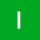Home
IT Knowledge
Inspiration
Languages
EN

# Java - convert camelCase to Sentence Case

0 points
Created by:illona
316

In this article, we would like to show you how to convert camelCase to sentence case in Java.

## 1. Simple solution

In this example, we use some string methods to convert camelCase to Sentence Case such as:

• `replaceAll()` with regex - to replace each capital letter with the same letter preceded by space character,
• `substring(0, 1)` - to get only the first letter from the string,
• `substring(1)` - to add whole string except the first character,
• `toUpperCase()` - to convert letters to upper case.
``````public class Example {

static Object sentenceCase(String text) {
if (!text.equals("")) {
String result = text.replaceAll("([A-Z])", " \$1");
return result.substring(0, 1).toUpperCase() + result.substring(1).toLowerCase();
}
return null;
}

public static void main(String[] args) {
String text = "mySampleText";
System.out.println(sentenceCase(text));  // My sample text
}
}``````

## 2. Optimal solution

This example present more complex but optimal solution.

When we want to reduce the number of compile function calls of the pattern class, we can explicitly create a pattern and then perform `matcher` and `replaceAll()` on it.

``````import java.util.regex.Matcher;
import java.util.regex.Pattern;

public class Example {

static final Pattern CAPITAL_LETTER_PATTERN = Pattern.compile("([A-Z])");

static Object sentenceCase(String text) {
if (text == null || text.isEmpty()) {
return text;
}
Matcher matcher = CAPITAL_LETTER_PATTERN.matcher(text);
String result = matcher.replaceAll(" \$1");
return result.substring(0, 1).toUpperCase() + result.substring(1).toLowerCase();
}

public static void main(String[] args) {
String text = "mySampleText";
System.out.println(sentenceCase(text));  // My sample text
}
}``````